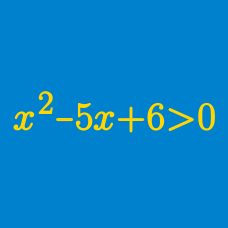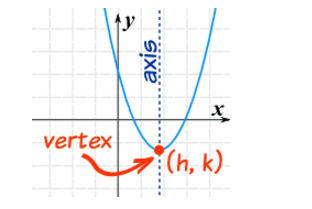Algebra

# Polynomial Inequalities: Level 3 Challenges

$\big(n^2-2\big)\big(n^2-20\big)<0$

How many integers $n$ satisfy the inequality above?

Over the ranges

\begin{aligned} -1 \leq & v & \leq 1 \\ -2 \leq & u & \leq -0.5 \\ -2 \leq & z & \leq -0.5 \\ \end{aligned}

what is the range of $w = \frac {vz}{u}$?

If $x$ is an integer that satisfies the inequality

$9 < x ^2 < 99,$

find the difference between the maximum and minimum possible values of $x.$

As $x$ and $y$ ranges over all real values, what is the minimum value of

$(15x + 30y + 20)^2 + (20x + 40y + 15)^2?$Given $y=\frac{2x}{1+x^{2}}$, where $x$ and $y$ are real numbers, what is the range of $y^{2}+y-2$?

×